# 句法分析技术1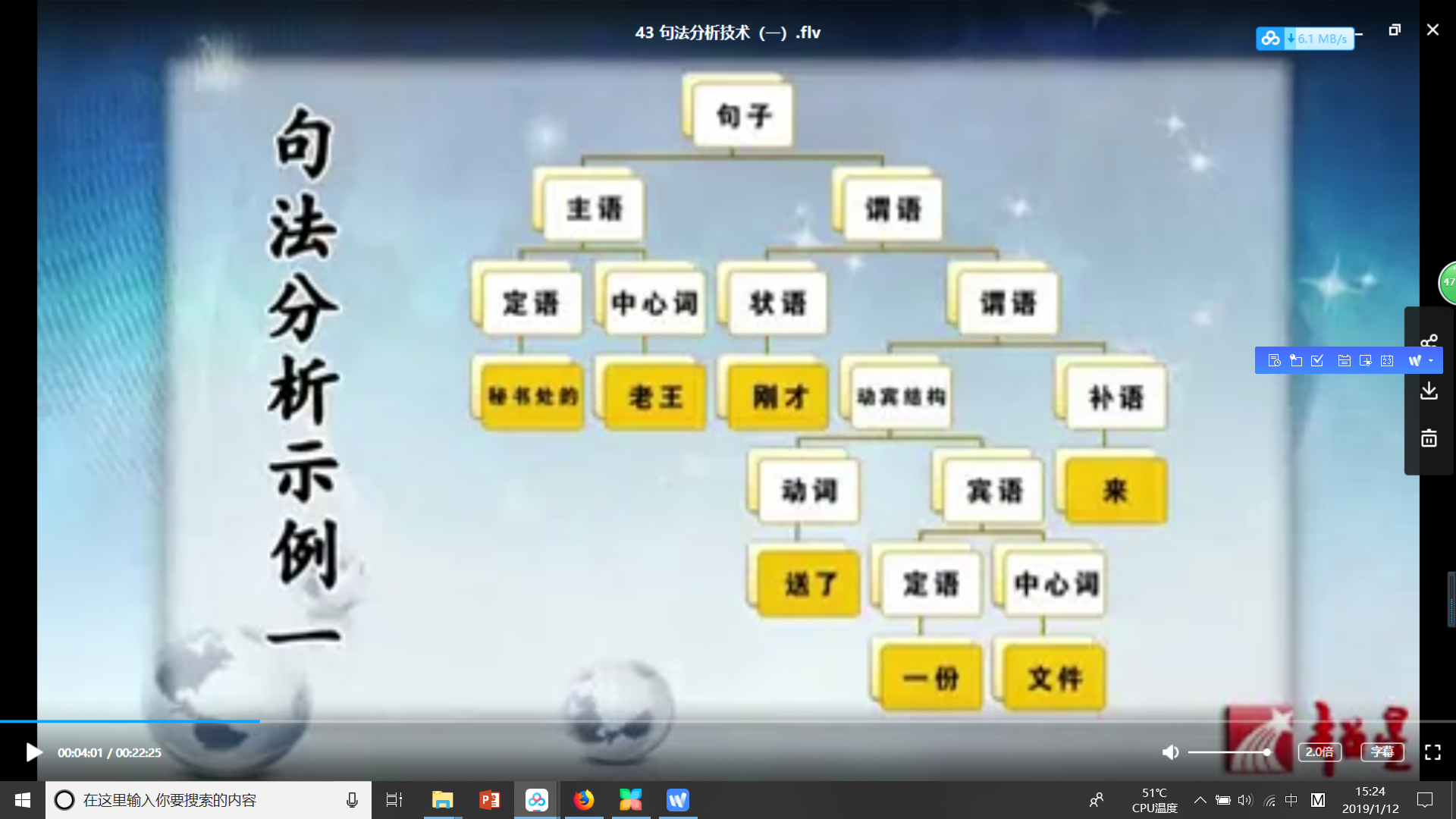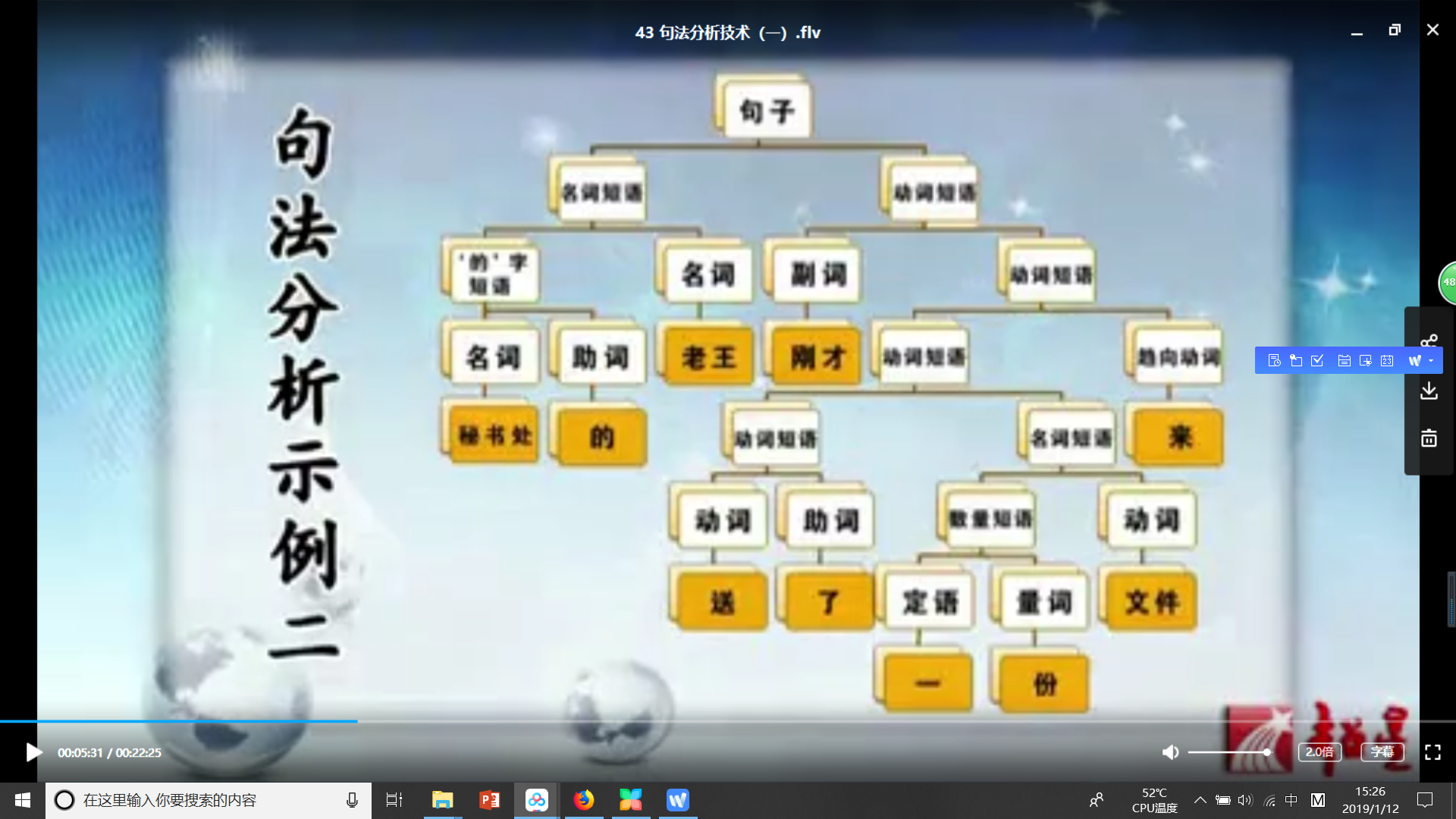# 句法分析技术2

$G=（N，\sum,P,S）$是一个文法，
$\alpha->\beta\in P$
0型文法：对 $\alpha->\beta 不作任何限制$
I型文法： $|\alpha|\leq|\beta|$
II型文法： $上下文无关文法，\alpha \in N$
III型文法：正则文法。

1）位置无关2）上下文无关3）祖先无关。

PCFG的三个基本问题。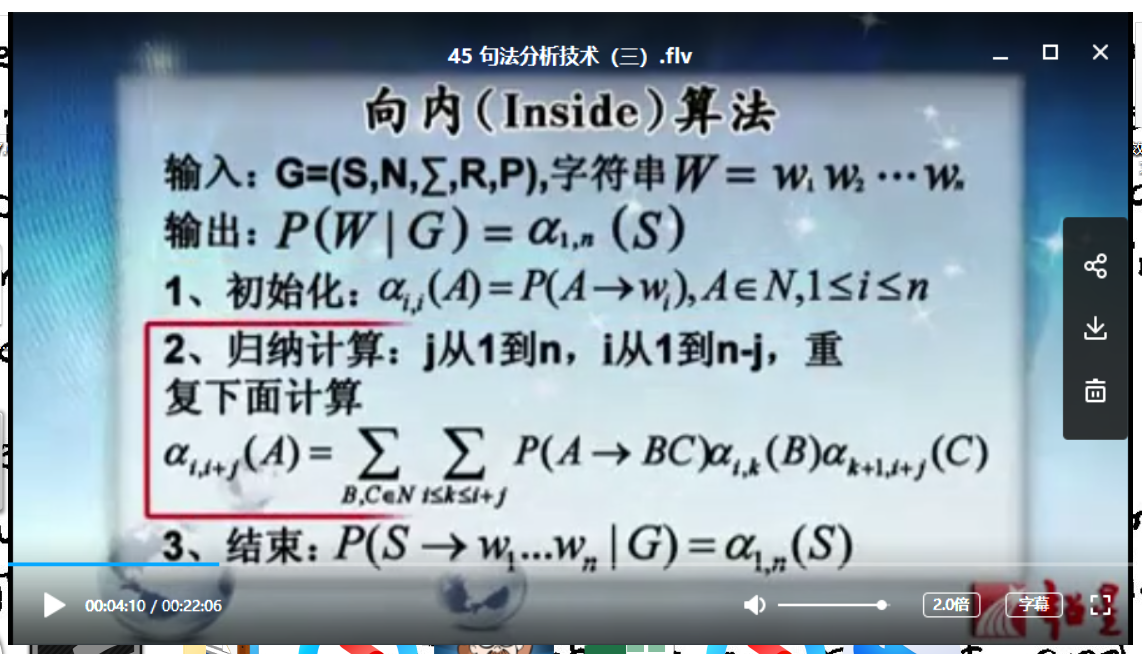# 句法分析技术3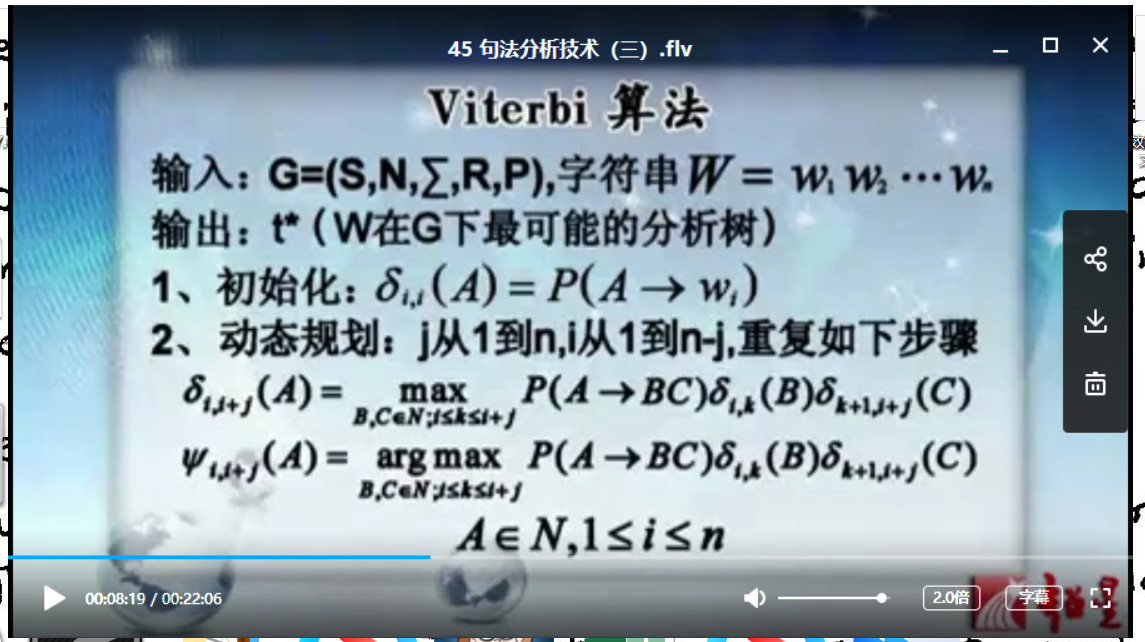某一部推导，对应于几个规则，开始推导，做出结果。

$\alpha_{ij}(A)=P(W_i,W_j|A),i<j$
$=\sum_{B,C,\in R}P(W_i,W_j,B,W_{r+1}..W_j,C|A)$
$\alpha_{i,j}=P(A->W_i)i=j$

# 句法分析技术4

S-k,r,c,p.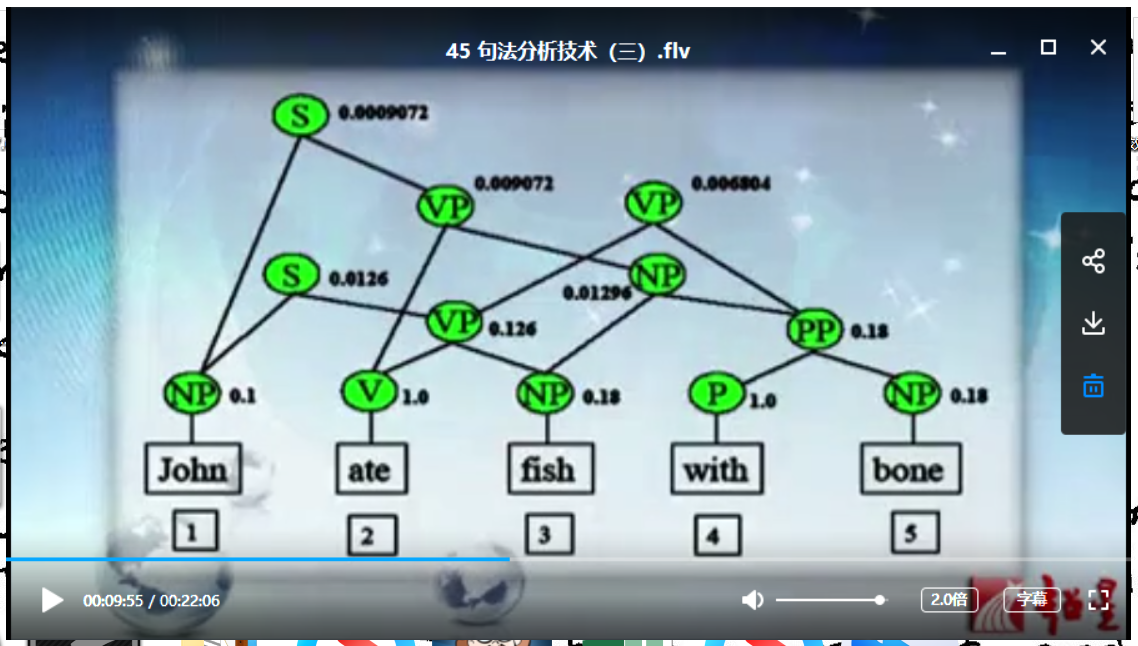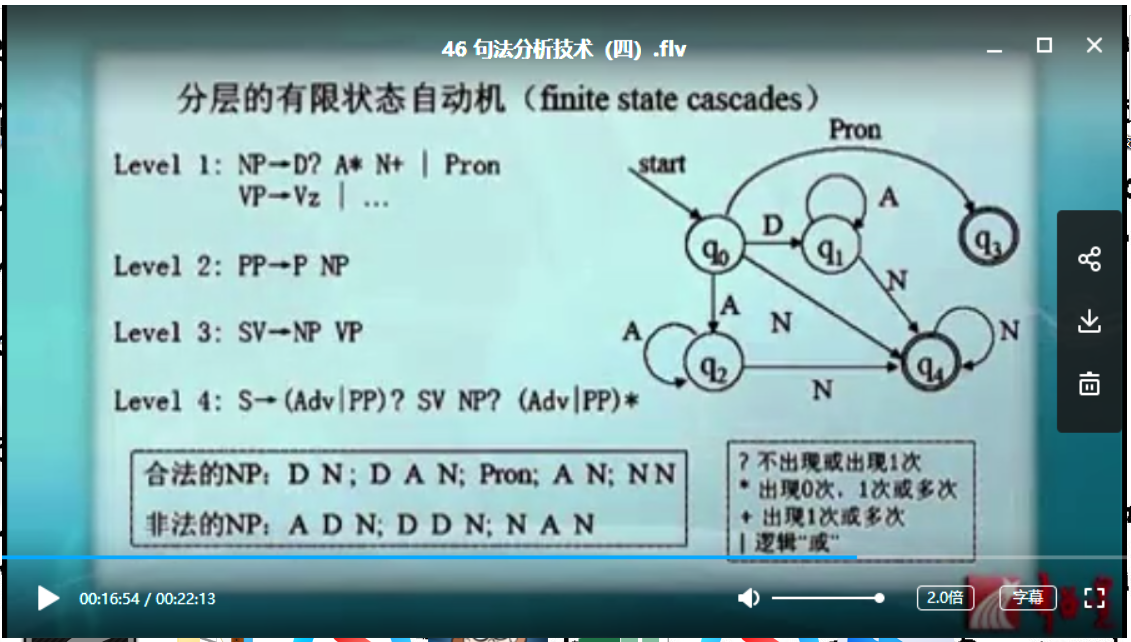# 句法分析技术5